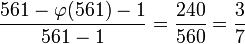# 561

## Summary

### Factorization

561 is a square-free number with prime factors 3, 11, and 17. The prime factorization is: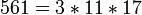### Properties and families

Property or family Parameter values First few numbers satisfying the property Proof of satisfaction/membership/containment
Carmichael number (also called absolute pseudoprime) first Carmichael number 561, 1105, 1729, 2465, 2821, 6601, [SHOW MORE]View list on OEIS The universal exponent is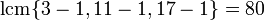which divides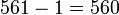Poulet number (Fermat pseudoprime to base 2) second Poulet number 341, 561, 645, 1105, 1387, 1729, 1905, 2047, [SHOW MORE] View list on OEIS follows from its being an odd Carmichael number.

## Arithmetic functions

Function Value Explanation
Euler totient function 320 The Euler totient function is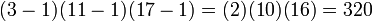.
universal exponent 80 The universal exponent is the least common multiple of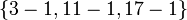, which is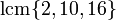and equals 80.
Note that 561 is a Carmichael number precisely because the universal exponent divides 561 - 1 = 560.
Mobius function -1 The number is a square-free number and it has an odd number of prime divisors (3 prime divisors).
divisor count function 8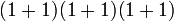where the first 1s in each sum denote the multiplicities of the prime divisors.
largest prime divisor 17
largest prime power divisor 17

## Structure of integers mod 561

### Abstract structure

The discussion here about the multiplicative group of integers modulo n relies on some background facts from group theory.
FACTS ABOUT MULTIPLICATIVE GROUPS: Classification of natural numbers for which the multiplicative group is cyclic | Characterization of multiplicative group of integers modulo n
GENERAL FACTS ABOUT GROUPS: order of direct product is product of orders, exponent of direct product is lcm of exponents

By the Chinese remainder theorem, we have the ring decomposition: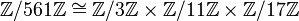For the multiplicative group, this gives: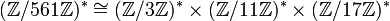All groups on the right side are cyclic, and we get:This group has order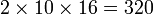(the order of the multiplicative group is termed the Euler totient function and denoted, so this is saying that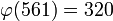).

The exponents of the factor groups are 2, 10, and 16 respectively, hence the exponent of the whole group is the lcm of these, which is 80. The exponent of the multiplicative group is termed the universal exponent and denoted, so this is saying that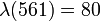).

## Primality testing

### Summary

Primality test Relies on ... Total number of candidate bases Number of bases wih gcd more than 1 Number of witnesses that are relatively prime Number of liars that are relatively prime Total number of witnesses Probability of picking witness Probability of picking liar Conditional probability of picking witness for relatively prime Conditional probability of picking liar for relatively prime
Fermat primality test Fermat's little theorem 560 240 0 320 240 240/560 = 3/7 4/7 0 1
Solovay-Strassen primality test Euler's criterion 560 240  ?  ?  ?  ?  ?  ?  ?
Miller-Rabin primality test 560 240  ?  ?  ?  ?  ?  ?  ?

### Fermat primality test

Further information: Fermat primality test

561 is a Fermat pseudoprime to base(or equivalently,is a Fermat liar for) if the order ofmod 3 divides 560, the order ofmod 11 divides 560, and the order ofmod 17 divides 560. Each of these conditions holds for allrelatively prime to 561, because each of the numbers 3-1,11-1, 17-1 divides 560. Another way of putting this is that the lcm of these, which is the universal exponent, divides 560.

Thus, 561 is a Fermat pseudoprime to every base relatively prime to it (or equivalently, every number relatively prime to 561 is a Fermat liar for 561). Numbers with this property are termed Carmichael numbers or absolute pseudoprimes. Thus, 561 is a Carmichael number. In fact, it is the smallest Carmichael number.

This means that the probability of success for a single iteration of the Fermat primality test is the probability that a randomly chosen element from 1 to 560 has a common factor with 561. This is given by: